# Plotting

Author

Marie-Hélène Burle

This section focuses on plotting in R with the package ggplot2 from the tidyverse.

## The data

R comes with a number of datasets. You can get a list by running `data()`. The `ggplot2` package provides additional ones. We will use the `mpg` dataset from `ggplot2`.

To access the data, let’s load the package:

``library(ggplot2)``

Here is what that dataset looks like:

``mpg``
``````# A tibble: 234 × 11
manufacturer model      displ  year   cyl trans      drv     cty   hwy fl
<chr>        <chr>      <dbl> <int> <int> <chr>      <chr> <int> <int> <chr>
1 audi         a4           1.8  1999     4 auto(l5)   f        18    29 p
2 audi         a4           1.8  1999     4 manual(m5) f        21    29 p
3 audi         a4           2    2008     4 manual(m6) f        20    31 p
4 audi         a4           2    2008     4 auto(av)   f        21    30 p
5 audi         a4           2.8  1999     6 auto(l5)   f        16    26 p
6 audi         a4           2.8  1999     6 manual(m5) f        18    26 p
7 audi         a4           3.1  2008     6 auto(av)   f        18    27 p
8 audi         a4 quattro   1.8  1999     4 manual(m5) 4        18    26 p
9 audi         a4 quattro   1.8  1999     4 auto(l5)   4        16    25 p
10 audi         a4 quattro   2    2008     4 manual(m6) 4        20    28 p
class
<chr>
1 compact
2 compact
3 compact
4 compact
5 compact
6 compact
7 compact
8 compact
9 compact
10 compact
# ℹ 224 more rows``````

`?mpg` will give you information on the variables. In particular:

• `displ` contains data on engine displacement (a measure of engine size and thus power) in litres (L).
• `hwy` contains data on fuel economy while driving on highways in miles per gallon (mpg).
• `drv` represents the type of drive train (front-wheel drive, rear wheel drive, 4WD).
• `class` represents the type of car.

We are interested in the relationship between engine size and fuel economy and see how the type of drive train and/or the type of car might affect this relationship.

## Base R plotting

R contains built-in plotting capability thanks to the `plot()` function.

A basic version of our plot would be:

``````plot(
mpg\$displ,
mpg\$hwy,
main = "Fuel consumption per engine size on highways",
xlab = "Engine size (L)",
ylab = "Fuel economy (mpg) on highways"
)``````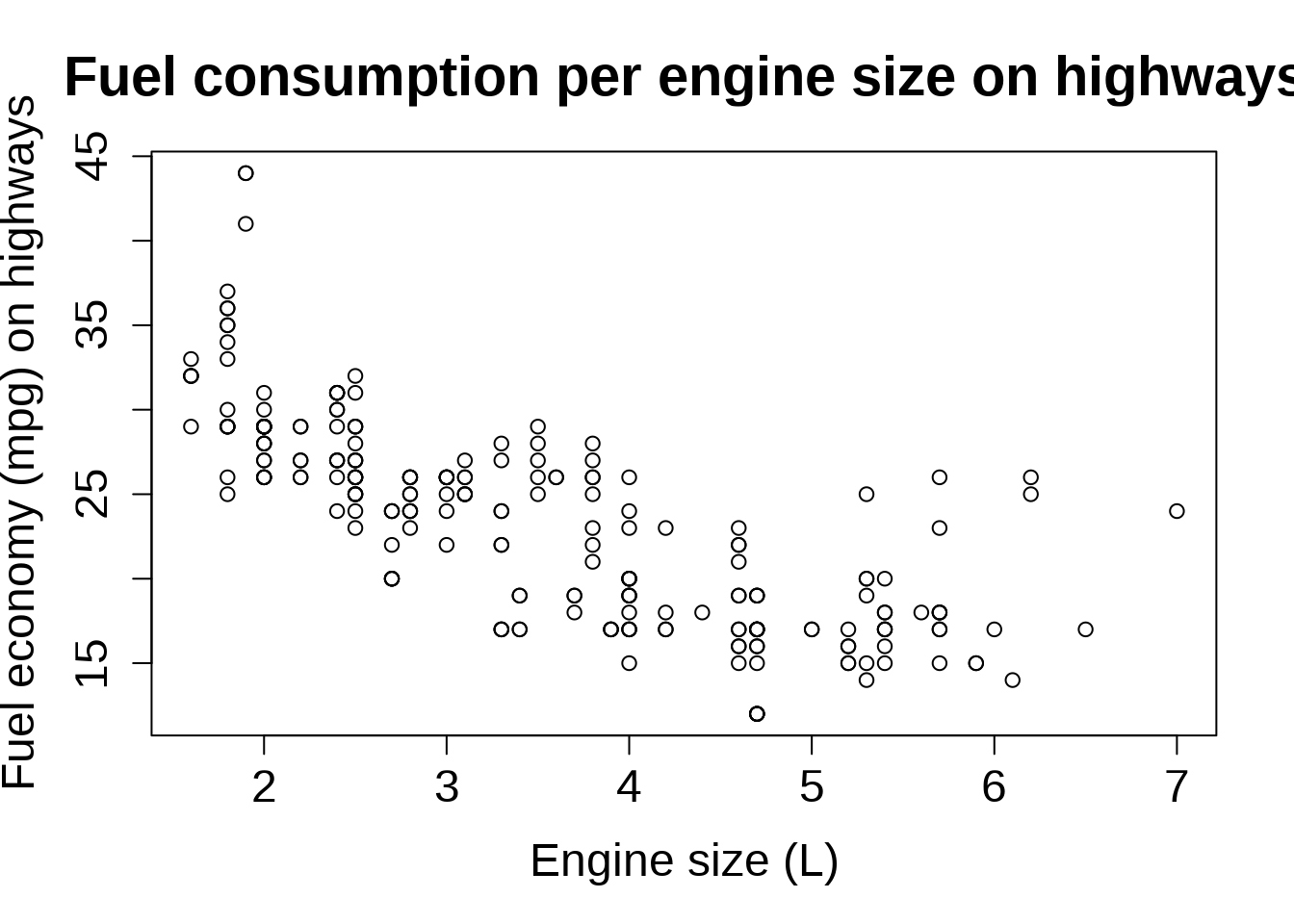## Grammar of graphics

Leland Wilkinson developed the concept of grammar of graphics in his 2005 book The Grammar of Graphics. By breaking down statistical graphs into components following a set of rules, any plot can be described and constructed in a rigorous fashion.

This was further refined by Hadley Wickham in his 2010 article A Layered Grammar of Graphics and implemented in the package `ggplot2` (that’s what the 2 “g” stand for in “ggplot”).

`ggplot2` has become the dominant graphing package in R. Let’s see how to construct a plot with this package.

## Plotting with `ggplot2`

You can find the `ggplot2` cheatsheet here.

### The Canvas

The first component is the data:

``ggplot(data = mpg)``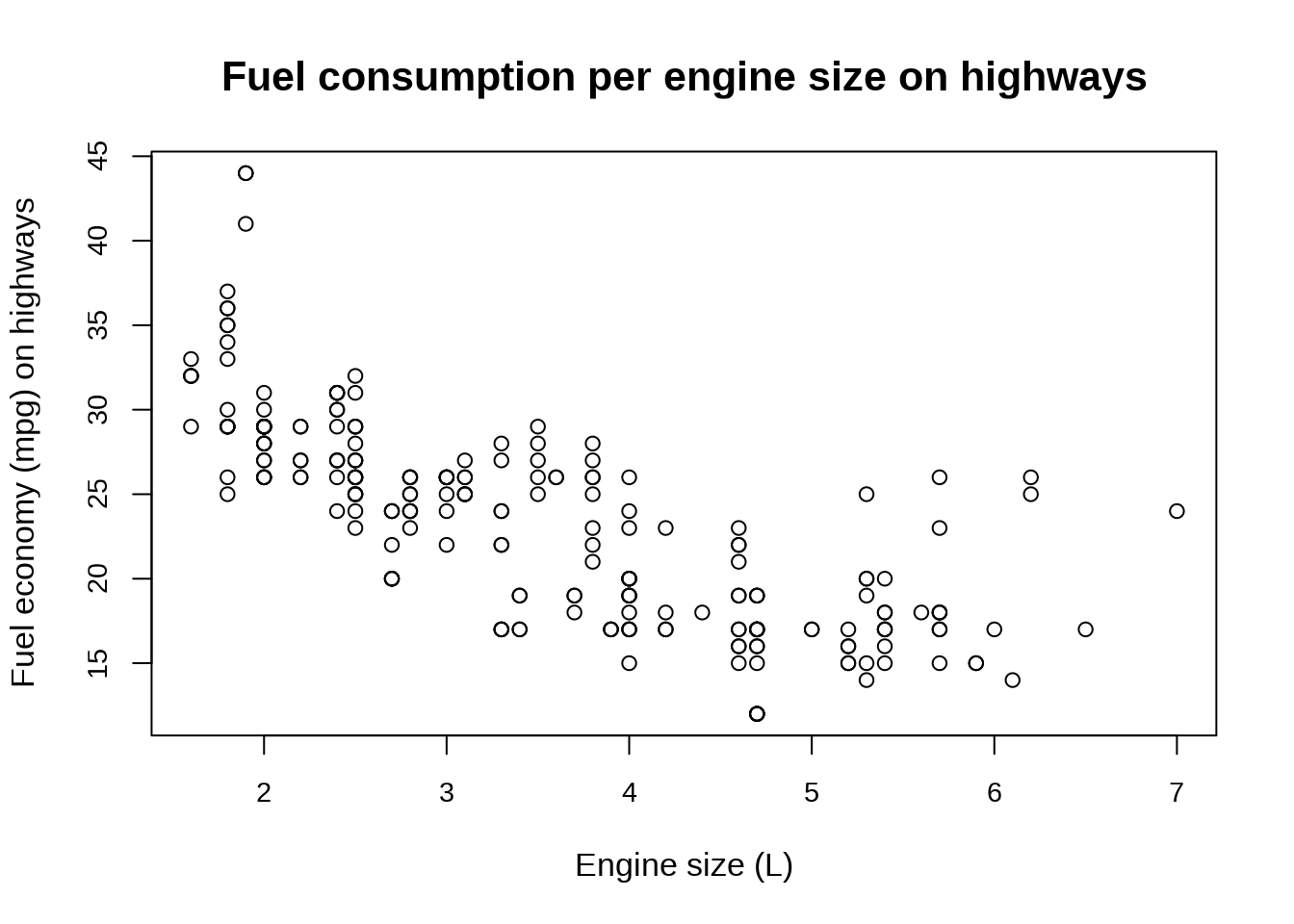This can be simplified into `ggplot(mpg)`.

The second component sets the way variables are mapped on the axes. This is done with the `aes()` (aesthetics) function:

``ggplot(data = mpg, mapping = aes(x = displ, y = hwy))``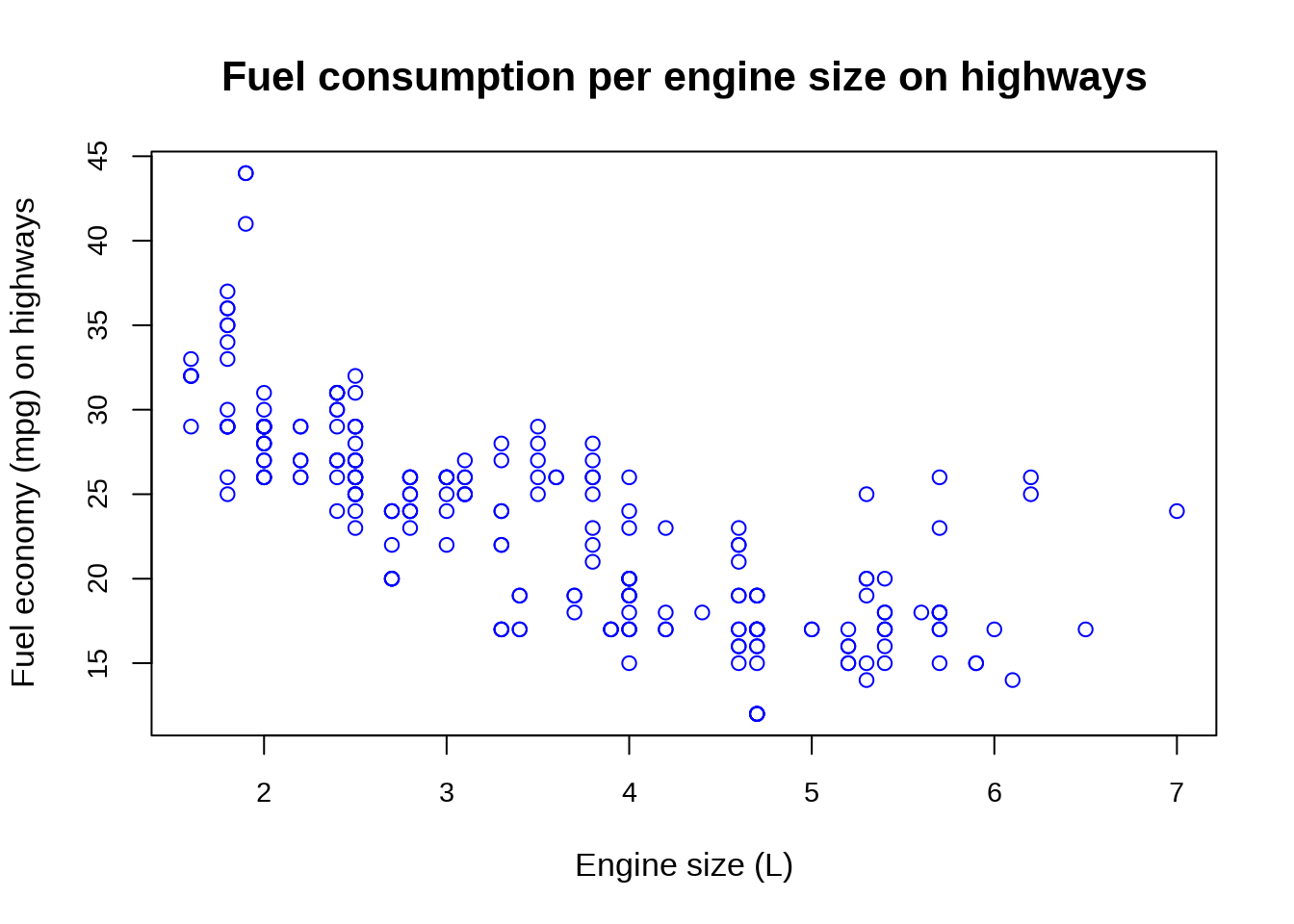This can be simplified into `ggplot(mpg, aes(x = displ, y = hwy))`.

### Geometric representations of the data

Onto this canvas, we can add “geoms” (geometrical objects) representing the data. The type of “geom” defines the type of representation (e.g. boxplot, histogram, bar chart).

To represent the data as a scatterplot, we use the `geom_point()` function:

``````ggplot(mpg, aes(x = displ, y = hwy)) +
geom_point()``````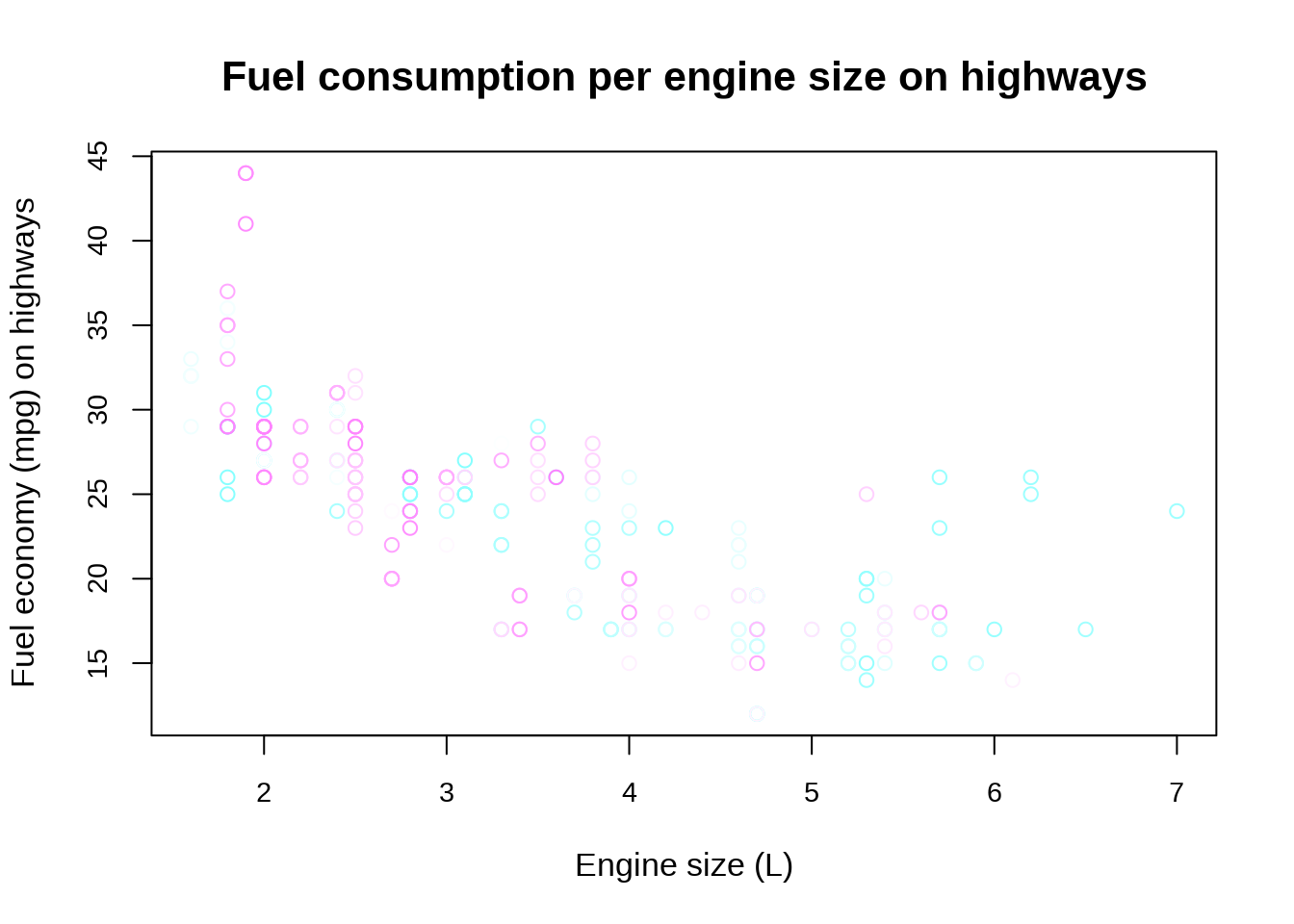We can colour-code the points in the scatterplot based on the `drv` variable, showing the lower fuel efficiency of 4WD vehicles:

``````ggplot(mpg, aes(x = displ, y = hwy)) +
geom_point(aes(color = drv))``````Or we can colour-code them based on the `class` variable:

``````ggplot(mpg, aes(x = displ, y = hwy)) +
geom_point(aes(color = class))``````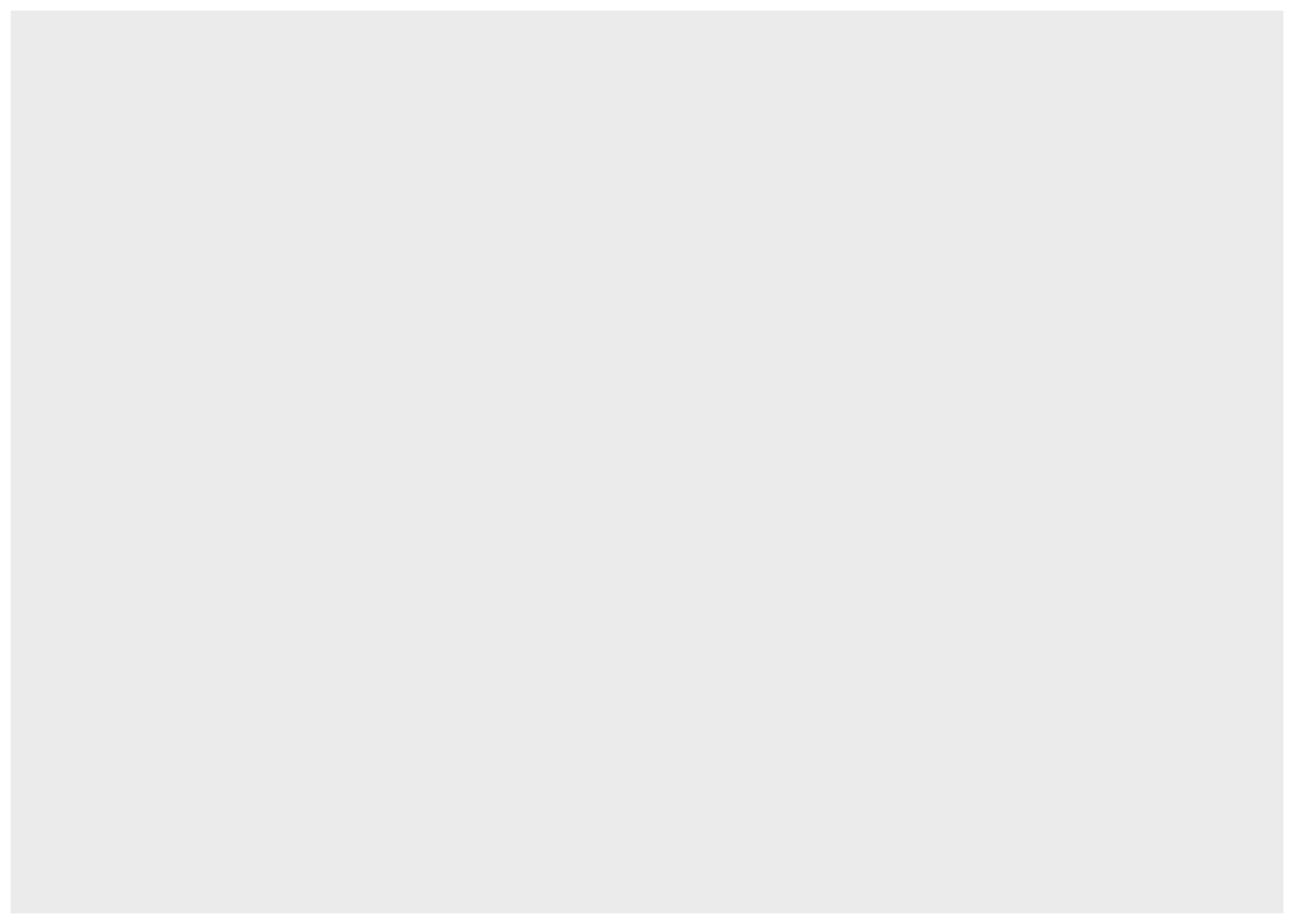Multiple “geoms” can be added on top of each other. For instance, we can add a smoothed conditional means function that aids at seeing patterns in the data with `geom_smooth()`:

``````ggplot(mpg, aes(x = displ, y = hwy)) +
geom_point(aes(color = class)) +
geom_smooth()``````
```geom_smooth()` using method = 'loess' and formula = 'y ~ x'``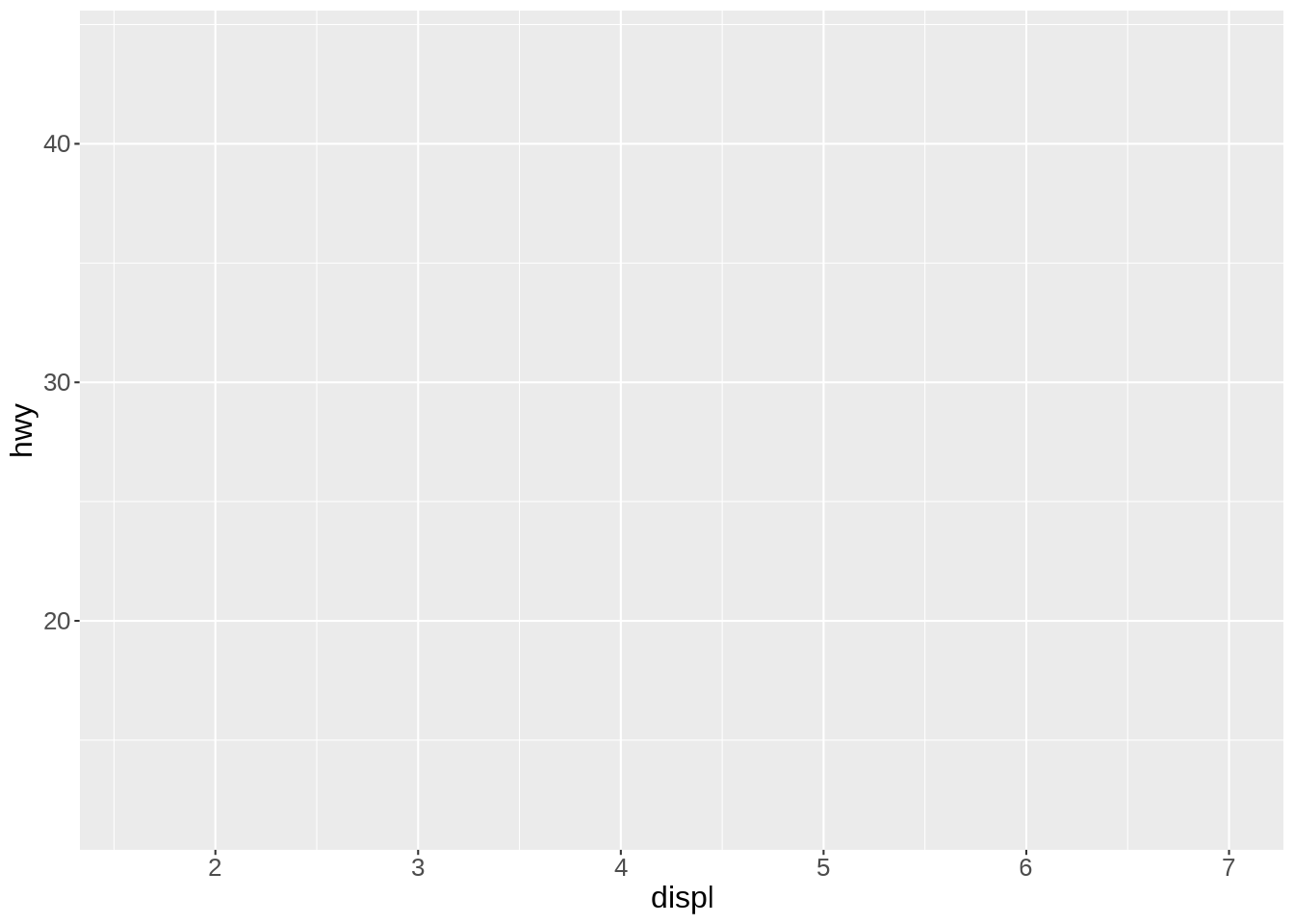Thanks to the colour-coding of the types of car, we can see that the cluster of points in the top right corner all belong to the same type: 2 seaters. Those are outliers with high power, yet high few efficiency due to their smaller size.

The default smoothing function uses the LOESS (locally estimated scatterplot smoothing) method, which is a nonlinear regression. But maybe a linear model would actually show the general trend better. We can change the method by passing it as an argument to `geom_smooth()`:

``````ggplot(mpg, aes(x = displ, y = hwy)) +
geom_point(aes(color = class)) +
geom_smooth(method = lm)``````
```geom_smooth()` using formula = 'y ~ x'``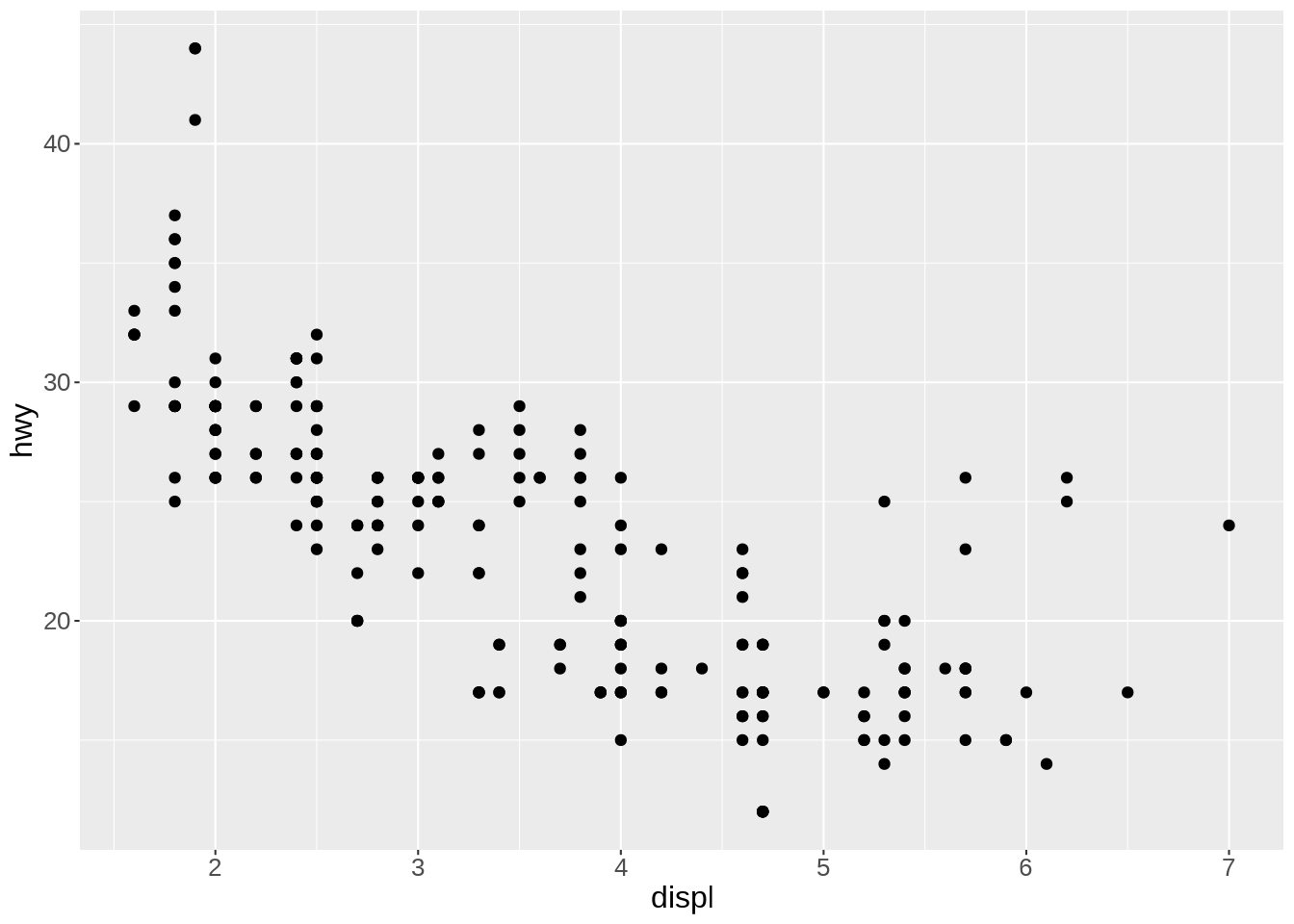Of course, we could apply the smoothing function to each class instead of the entire data. It creates a busy plot but shows that the downward trend remains true within each type of car:

``````ggplot(mpg, aes(x = displ, y = hwy, color = class)) +
geom_point(aes(color = class)) +
geom_smooth(method = lm)``````
```geom_smooth()` using formula = 'y ~ x'``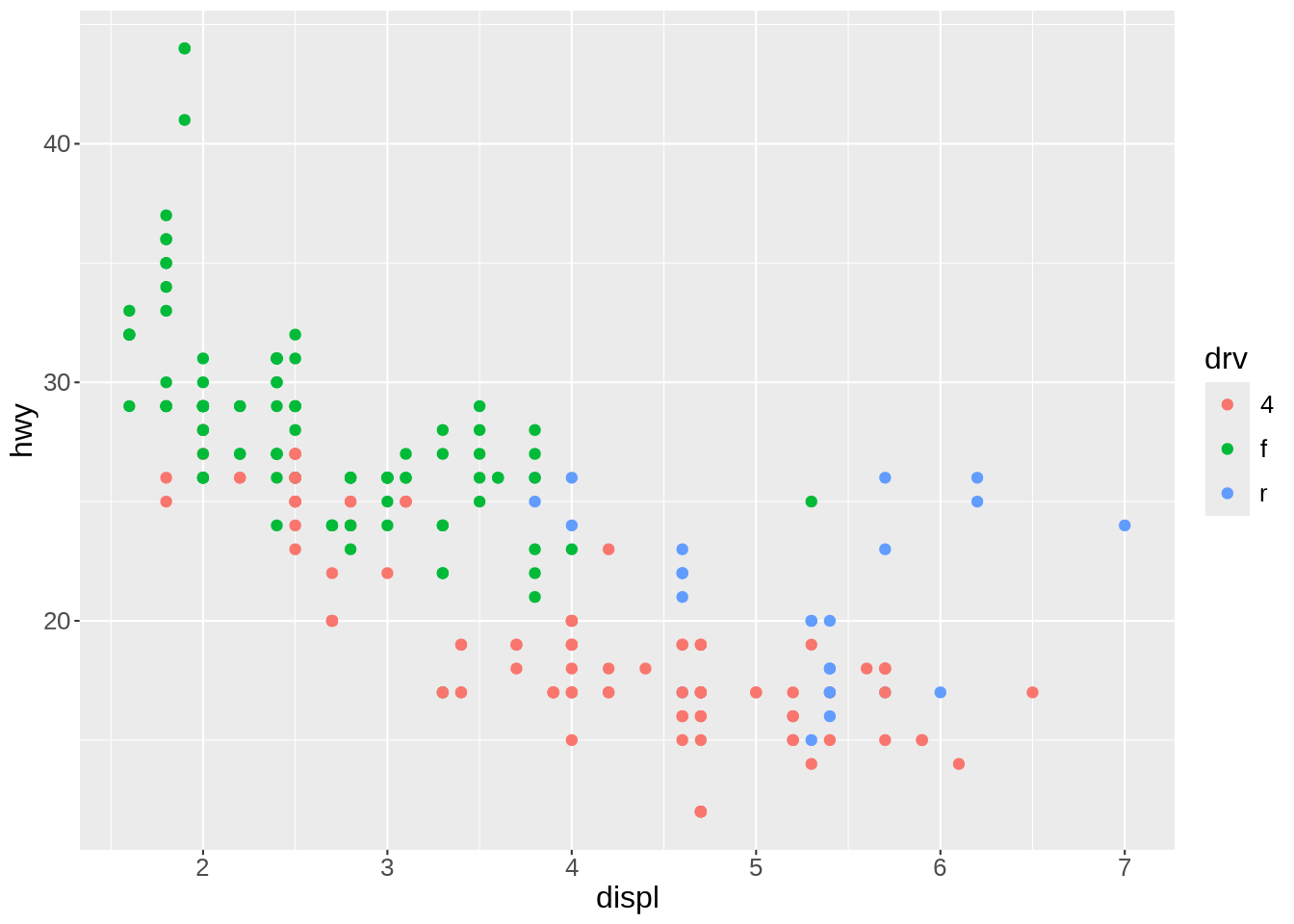Other arguments to `geom_smooth()` can set the line width, color, or whether or not the standard error (`se`) is shown:

``````ggplot(mpg, aes(x = displ, y = hwy)) +
geom_point(aes(color = class)) +
geom_smooth(
method = lm,
se = FALSE,
color = "#999999",
linewidth = 0.5
)``````
```geom_smooth()` using formula = 'y ~ x'``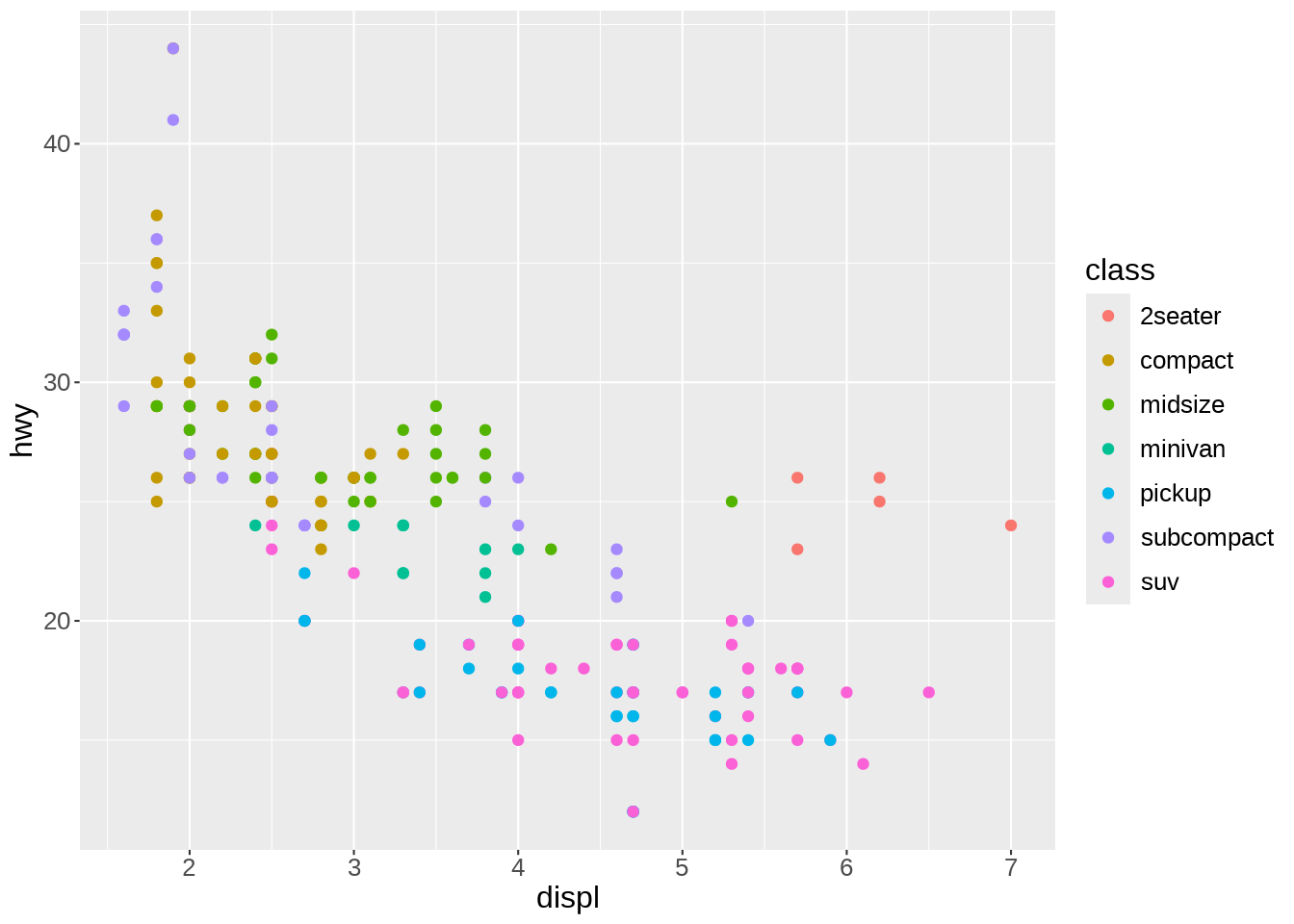### Colour scales

If we want to change the colour scale, we add another layer for this:

``````ggplot(mpg, aes(x = displ, y = hwy)) +
geom_point(aes(color = class)) +
scale_color_brewer(palette = "Dark2") +
geom_smooth(
method = lm,
se = FALSE,
color = "#999999",
linewidth = 0.5
)``````
```geom_smooth()` using formula = 'y ~ x'``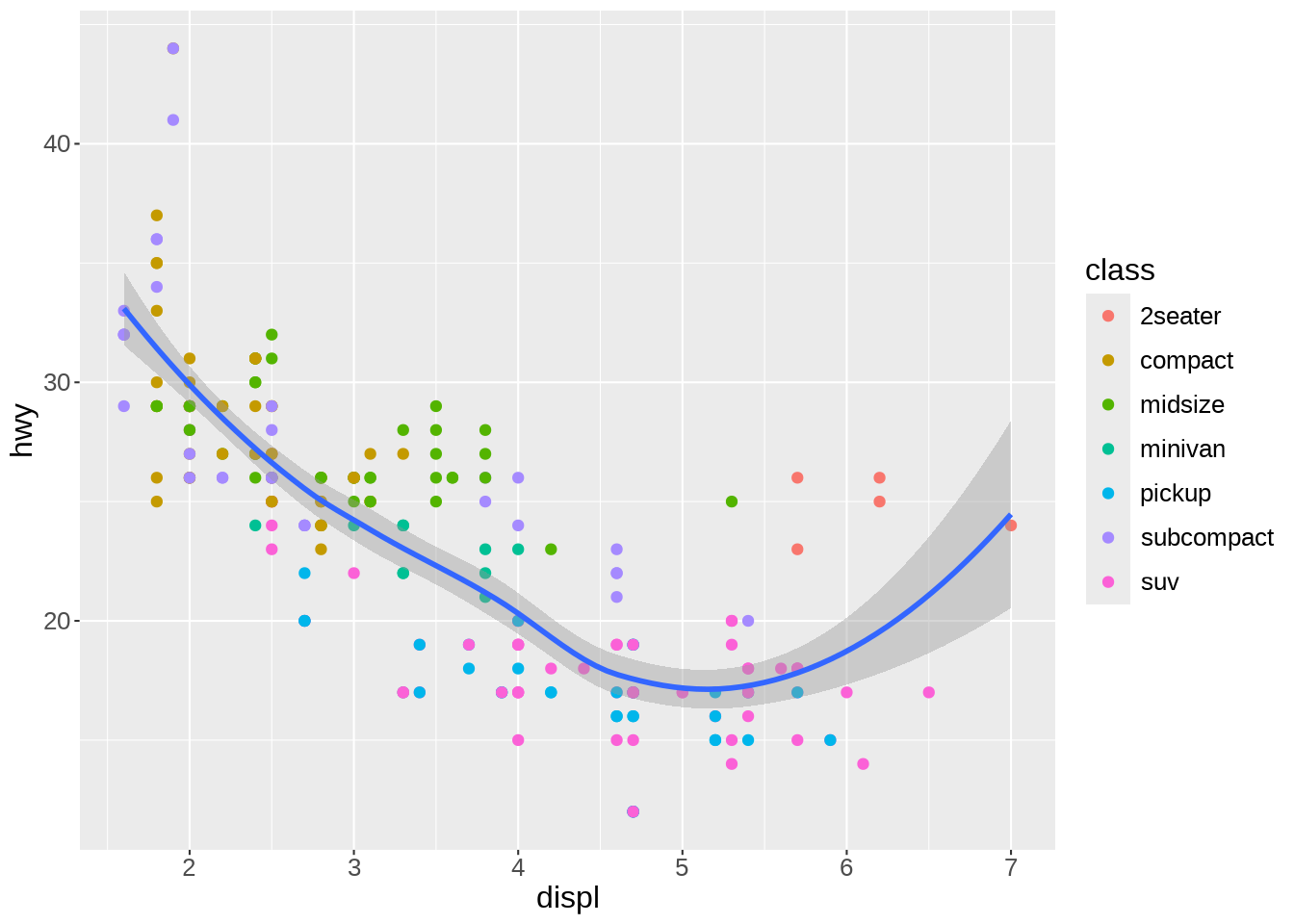`scale_color_brewer()`, based on color brewer 2.0, is one of many methods to change the color scale. Here is the list of available scales for this particular method: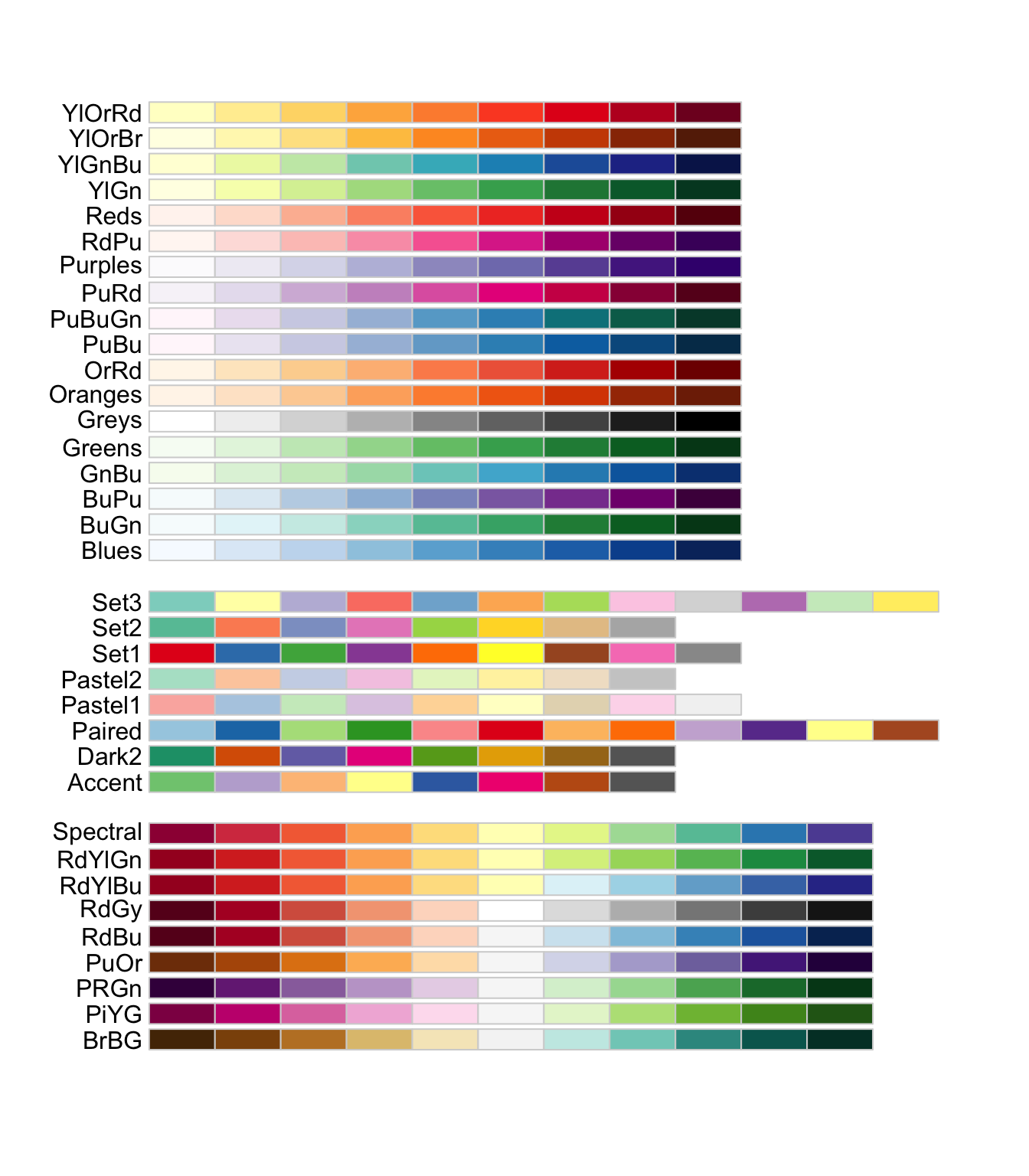### Labels

We can keep on adding layers. For instance, the `labs()` function allows to set title, subtitle, captions, tags, axes labels, etc.

``````ggplot(mpg, aes(x = displ, y = hwy)) +
geom_point(aes(color = class)) +
scale_color_brewer(palette = "Dark2") +
geom_smooth(
method = lm,
se = FALSE,
color = "#999999",
linewidth = 0.5
) +
labs(
title = "Fuel consumption per engine size on highways",
x = "Engine size (L)",
y = "Fuel economy (mpg) on highways",
color = "Type of car",
caption = "EPA data from https://fueleconomy.gov/"
)``````
```geom_smooth()` using formula = 'y ~ x'``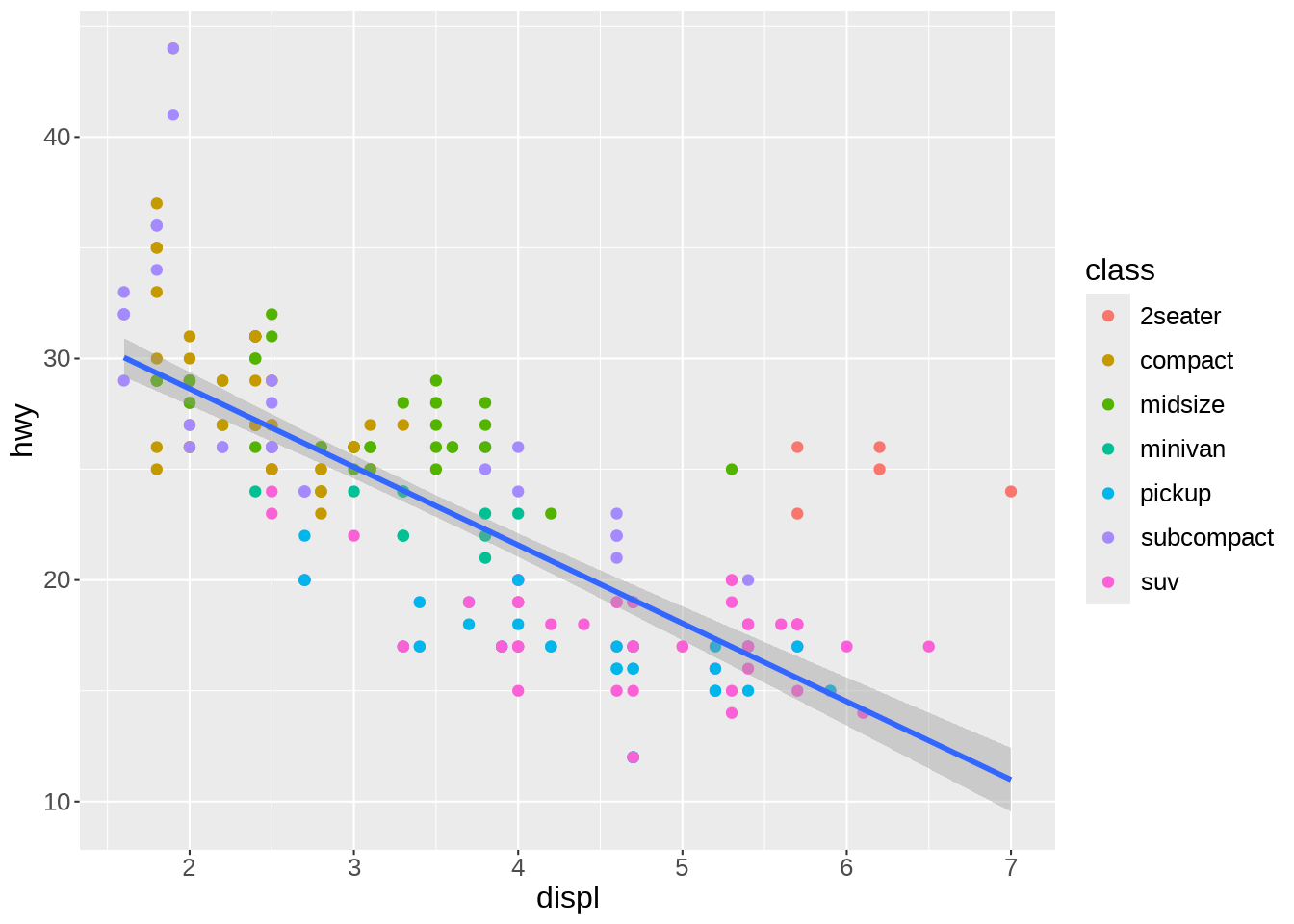### Themes

Another optional layer sets one of several preset themes.

Edward Tufte developed, amongst others, the principle of data-ink ratio which emphasizes that ink should be used primarily where it communicates meaningful messages. It is indeed common to see charts where more ink is used in labels or background than in the actual representation of the data.

The default `ggplot2` theme could be criticized as not following this principle. Let’s change it:

``````ggplot(mpg, aes(x = displ, y = hwy)) +
geom_point(aes(color = class)) +
scale_color_brewer(palette = "Dark2") +
geom_smooth(
method = lm,
se = FALSE,
color = "#999999",
linewidth = 0.5
) +
labs(
title = "Fuel consumption per engine size on highways",
x = "Engine size (L)",
y = "Fuel economy (mpg) on highways",
color = "Type of car",
caption = "EPA data from https://fueleconomy.gov/"
) +
theme_classic()``````
```geom_smooth()` using formula = 'y ~ x'``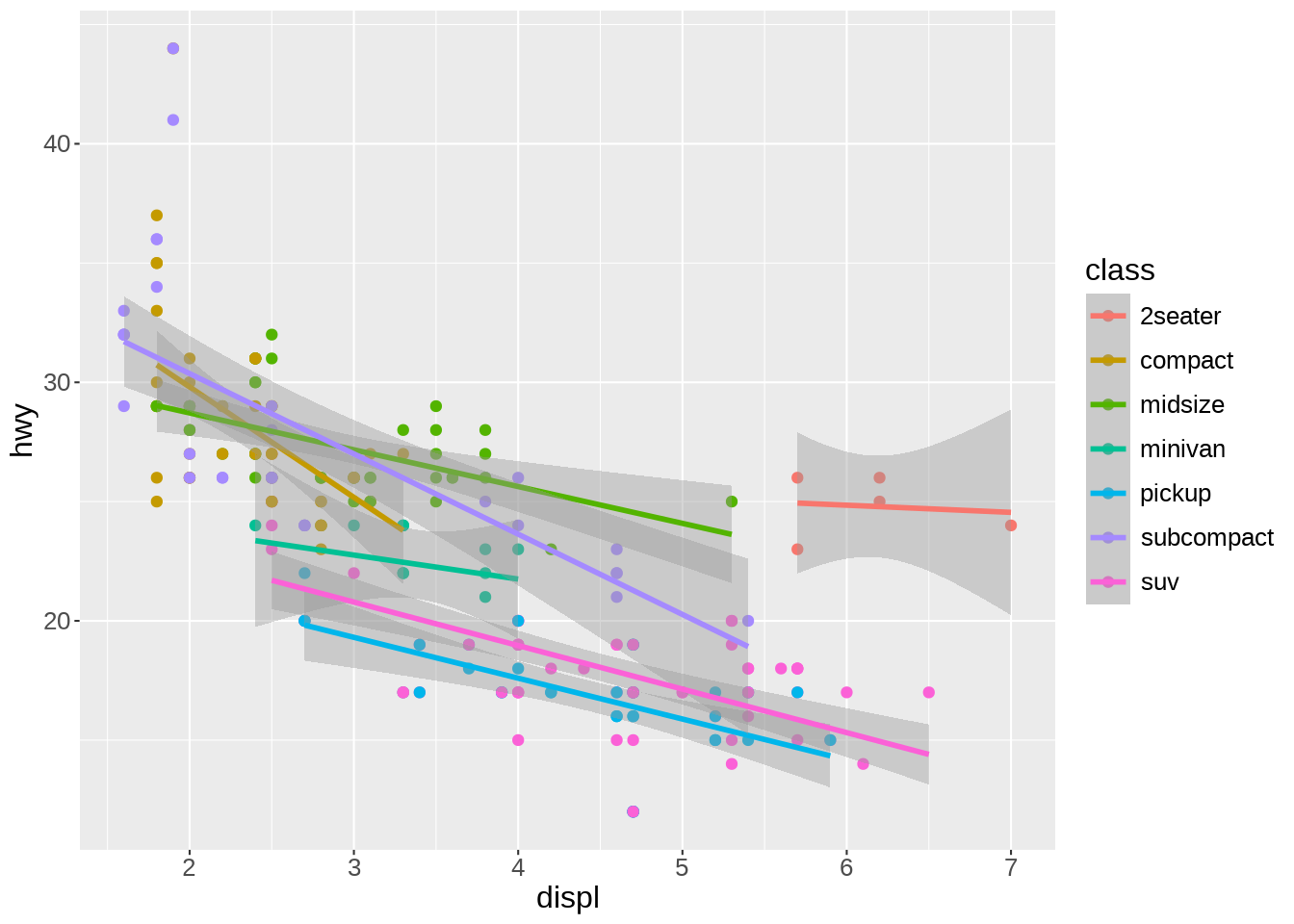The `theme()` function allows to tweak the theme in any number of ways. For instance, what if we don’t like the default position of the title and we’d rather have it centered?

``````ggplot(mpg, aes(x = displ, y = hwy)) +
geom_point(aes(color = class)) +
scale_color_brewer(palette = "Dark2") +
geom_smooth(
method = lm,
se = FALSE,
color = "#999999",
linewidth = 0.5
) +
labs(
title = "Fuel consumption per engine size on highways",
x = "Engine size (L)",
y = "Fuel economy (mpg) on highways",
color = "Type of car",
caption = "EPA data from https://fueleconomy.gov/"
) +
theme_classic() +
theme(plot.title = element_text(hjust = 0.5))``````
```geom_smooth()` using formula = 'y ~ x'``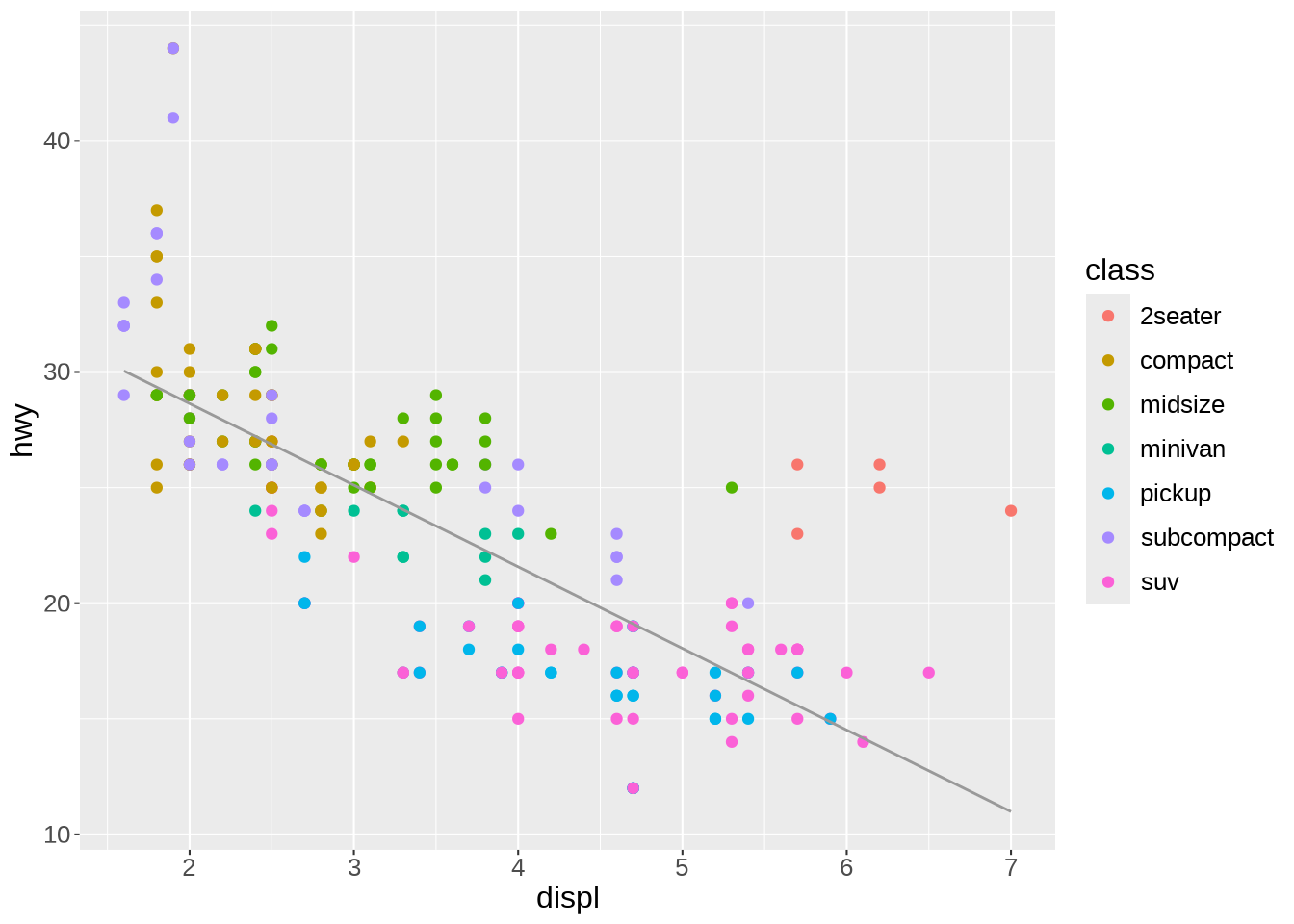Many things can be changed thanks to the `theme()` function. For instance, we can move the legend to give more space to the actual graph:

``````ggplot(mpg, aes(x = displ, y = hwy)) +
geom_point(aes(color = class)) +
scale_color_brewer(palette = "Dark2") +
geom_smooth(
method = lm,
se = FALSE,
color = "#999999",
linewidth = 0.5
) +
labs(
title = "Fuel consumption per engine size on highways",
x = "Engine size (L)",
y = "Fuel economy (mpg) on highways",
color = "Type of car",
caption = "EPA data from https://fueleconomy.gov/"
) +
theme_classic() +
theme(plot.title = element_text(hjust = 0.5), legend.position = "bottom")``````
```geom_smooth()` using formula = 'y ~ x'``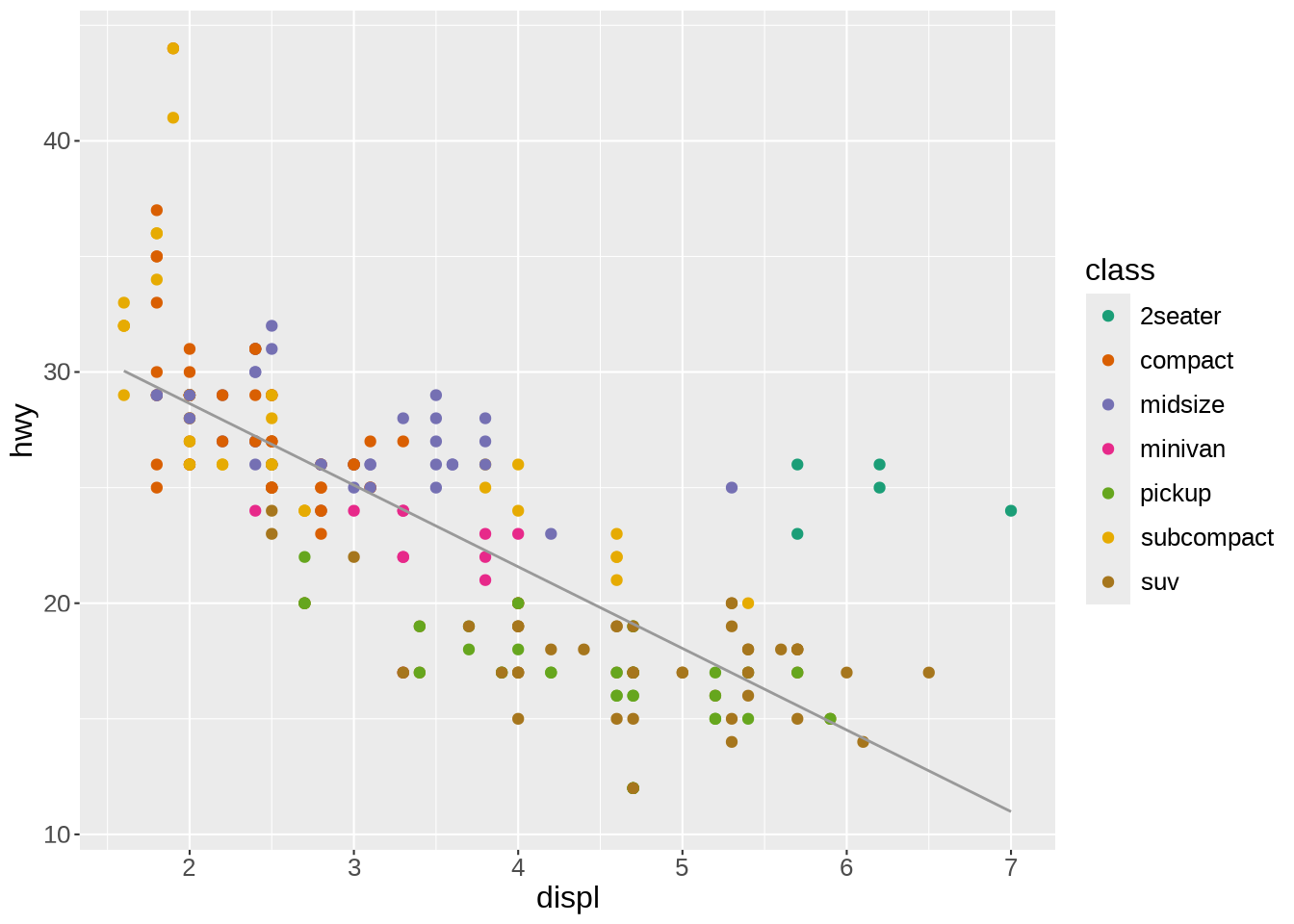As you could see, `ggplot2` works by adding a number of layers on top of each other, all following a standard set of rules, or “grammar”. This way, a vast array of graphs can be created by organizing simple components.

## `ggplot2` extensions

Thanks to its vast popularity, `ggplot2` has seen a proliferation of packages extending its capabilities.

### Combining plots

For instance the `patchwork` package allows to easily combine multiple plots on the same frame.

Let’s add a second plot next to our plot. To add plots side by side, we simply add them to each other. We also make a few changes to the labels to improve the plots integration:

``````library(patchwork)

ggplot(mpg, aes(x = displ, y = hwy)) +        # First plot
geom_point(aes(color = class)) +
scale_color_brewer(palette = "Dark2") +
geom_smooth(
method = lm,
se = FALSE,
color = "#999999",
linewidth = 0.5
) +
labs(
x = "Engine size (L)",
y = "Fuel economy (mpg) on highways",
color = "Type of car"
) +
theme_classic() +
theme(
plot.title = element_text(hjust = 0.5),
legend.position = c(0.7, 0.75),           # Better legend position
legend.background = element_rect(         # Add a frame to the legend
linewidth = 0.1,
linetype = "solid",
colour = "black"
)
) +
ggplot(mpg, aes(x = displ, y = hwy)) +      # Second plot
geom_point(aes(color = drv)) +
scale_color_brewer(palette = "Dark2") +
labs(
x = "Engine size (L)",
y = element_blank(),                      # Remove redundant label
color = "Type of drive train",
caption = "EPA data from https://fueleconomy.gov/"
) +
theme_classic() +
theme(
plot.title = element_text(hjust = 0.5),
legend.position = c(0.7, 0.87),
legend.background = element_rect(
linewidth = 0.1,
linetype = "solid",
colour = "black"
)
)``````
```geom_smooth()` using formula = 'y ~ x'``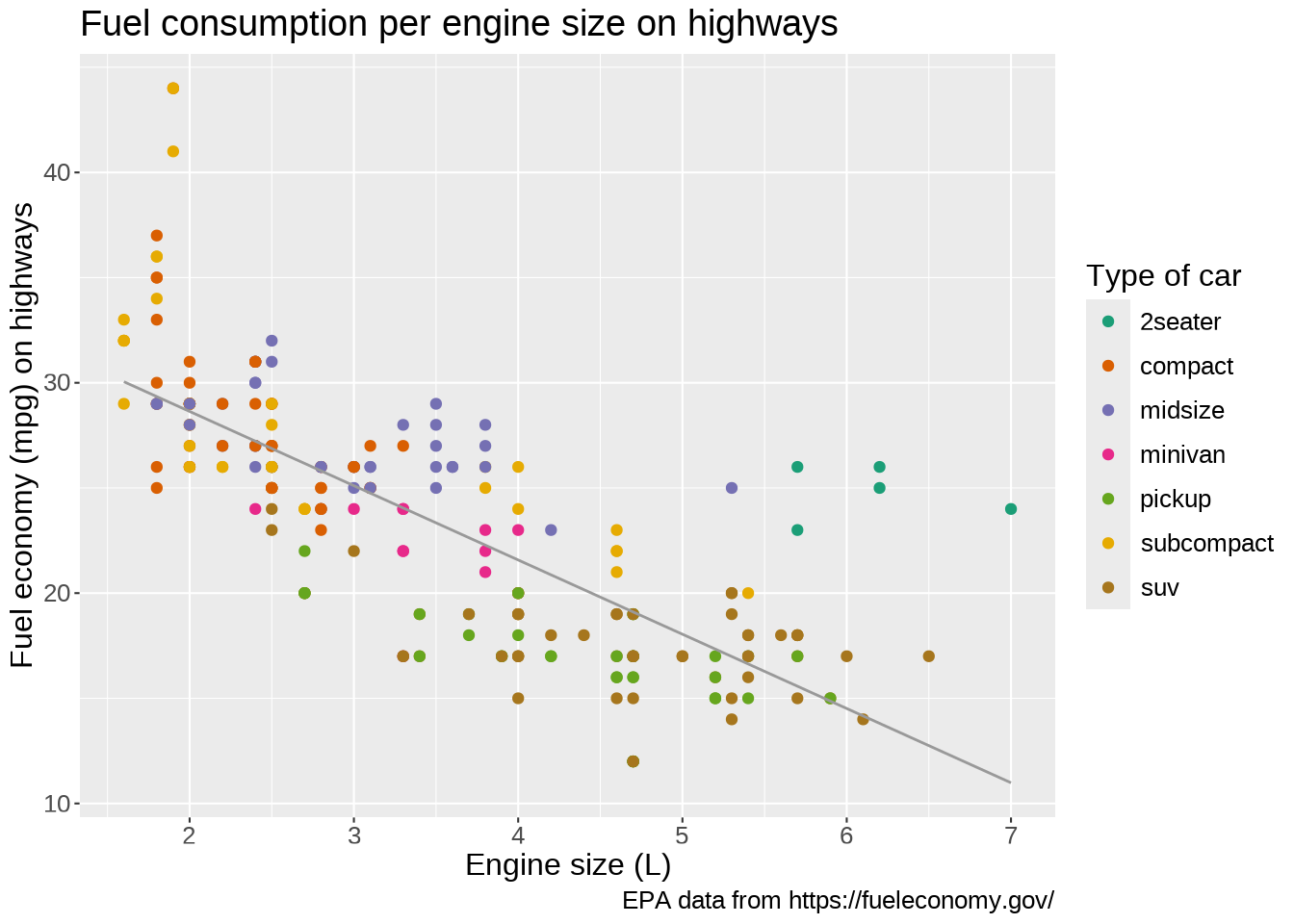### Extensions list

Another popular extension is the `gganimate` package which allows to create data animations.

A full list of extensions for `ggplot2` is shown below (here is the website):